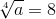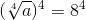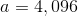# SAT II Math II : Other Mathematical Relationships

## Example Questions

### Example Question #1 : Other Mathematical Relationships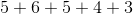Explanation:

In modulo 9 arithmetic, a number is congruent to the remainder of its division by 9.

Since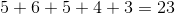and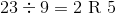,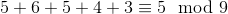,

making "5" the correct response.

### Example Question #2 : Other Mathematical Relationshipsvaries directly as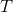and inversely asIf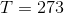and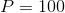, then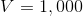.

To the nearest whole number, evaluateif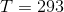and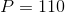.

Insufficient information is given to answer the question.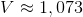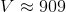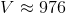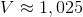Explanation:varies directly asand inversely as. This means that for some constant of variation,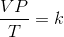Alternatively,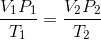We can substitute the initial conditions for thevariables on the left side and the final conditions for those on the right side, then solve for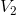: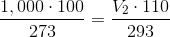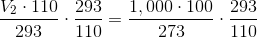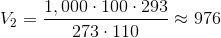### Example Question #3 : Other Mathematical Relationships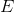varies directly as bothand the square of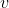.

If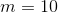and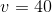, then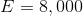Evaluateif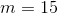and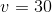.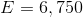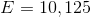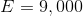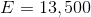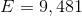Explanation:varies directly as bothand the square of. This means that for some constant of variation,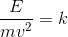.

Alternatively stated,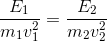.

We can substitute the initial conditions for the variables on the left side and the final conditions for those on the right side, then solve for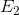: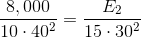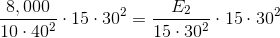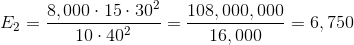### Example Question #4 : Other Mathematical Relationships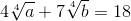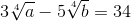Evaluate.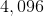The system has no solution.Explanation:

Rewrite the two equations by setting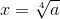and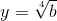and substituting: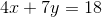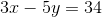The result is a two-by-two linear system in terms ofand:This can be solved, among other ways, using Gaussian elimination on an augmented matrix: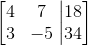Perform row operations until the left two columns show identity matrix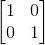. One possible sequence: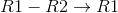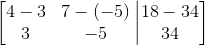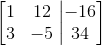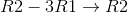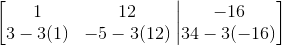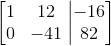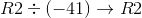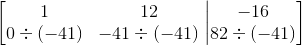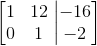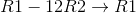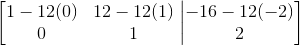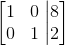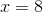and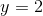. In the former equation, substitute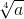back for, and raise both sides to the power of 4: